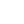# Definition

Minimum spanning tree is defined as given graph(G) which a set of vertexes and edges with weights. Find a path which connects all vertexes with minimum total weight of edges. There are two classic algorithms which are designed with greedy algorithm: Prim Algorithm and Kruskal Algorithm.

## Generice MST

``````A = {} // subset of some minimum spanning tree (V, E)
while A is not a minimum spanning tree
find edge(u, v) to that is safe to A
A = A union (u, v)``````

## Safe Edge

Safe edge is a edge that is safe to added into A which out destorying the invarient property that A is subet of some minimum spanning tree. In other words, when you add a edge into A which causes A cannot form MST in the future, the edge is not safe.

### Light Edge is safe

Let G=(V,E) be a connected, undirected graph with a real-valued weight function w defined on E. Let A be a subset of E that is included in some minimum spanning tree for G. Let (S,V-S) be any cut of G such that for any edge (u, v) in A, {u, v} S or {u, v} (V-S). Let (u,v) be a light edge crossing (S,V-S). Then, edge (u,v) is safe for A. (.... It just means finding a minimum weight edge which connects two parts of the graph, when it is cut then no vertexes are shared in two parts)

# Prim Algorithm

This algorithm is based on shortest path algorithm. It builds a set of A in the process and select the light edge connecting A to others.

Simple version

``````  A = {}
S = {r} // r is the random node you want to start with
Q = V - {r}
while Q is not empty
Find the minimum edge(u, v) so that u in A and v in Q
Add (u, v) in A, add v in S, and delete v from Q``````

Priortiy queue version The min-priority queue is implemented with binary heap. Use the key of the priority queue to keep track of the minimum distance from A to others. Note: Heap-Order: for every node v other than the root, key(v) >= key(parent(v))

``````    Store all V in priority queue Q
for each u in Q
key[u] = infinity
parent[u] = NUL
key[r] = 0
while (Q is not empty)
u = EXTRACT_MIN(Q)
// v belongs to others but not the buidling tree
// update its minimum distance if edge is smaller than its expected value before
if (v belongs to Q && key[v] > w(u,v) )
key[v] = w(u, v)
parent[v] = u``````

## Running time analysis

While loop executes V times EXTRACT_MIN(Q) : log V => V log V

The for loop (each v in adj[u]): total executes 2E times decrease key operation : log V => E log V

Overall running time: (V log V + E log V) = E log V // E always > V

# Kruskal Algorithm

Add the edges according to the increasing weight, as long as the operation will not violate the minimum tree property. It uses a disjoint set to keep track the grouping of vertexes, as long as the edge(u, v) where u and v are not in the same group, the edge is safe to be added.

``````  A = {} // tree under construction
Sort the edges of E by weights by increasing order
for each v in G
MAKE_SET(v) // every one belongs to its own group initially
for each edge(u, v) in E
if (FIND_SET(u) != FIND_SET(v))
A union (u, v)
UNION(u, v) // they are the same group now
return A``````

## Running time analysis

1. sort edges: E log E
2. for loop V times: MAKE_SET: log V
3. for loop execcutes E times FIND_SET and UNION: log V

Combine 2 & 3 we ge V+E log V => E log V => combine with (1) E log E Overall Running time: E log E

## Disjoint Set Implemented by array

Every child remember its parent index The parent remeber its size (-ve)

``````int Find(int element){
if (nodes[element] < 0)
return element;
else
return nodes[element] = Find(nodes[element]);
}

void UnionSet(int set1, int set2){
nodes[set1] += nodes[set2];
nodes[set2] = set1;
}

int Union(int element1, int element2){
int root1 = Find(element1);
int root2 = Find(element2);

if (root1 == root2) // reject if form a cycle
return 0;

if (nodes[root1] < nodes[root2]) // root1 has more elements than root2
UnionSet(root1, root2);
else
UnionSet(root2, root1);

return 1;
}``````

## Differene between Prim algorihtm and Kruskal algorithm

Prim requires a connected graph, Kruskal can work on unconnected group in which many MST can be formed. Every step in prim is a partial solution.

## Application of MST

MST algorihtm can be also used to build spanning tree based on each side

``````for (int i=0; i<m; i++){
// based on each side, try to build a spanning tree with bigger side
// compare their slimness
for (int j=1; j<=n; j++){
nodes[j] = -1;
}

Edge b = edges[i];
Union(b.u, b.v);

int largest = b.c;
int smallest = b.c;
for (int j=i+1; j<m; j++){
Edge c = edges[j];
Union(c.u, c.v);
}
}``````

k - cluster with mini distance between point = MST - k most expensive edges.